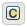Modify the Action Language for a Chart

Stateflow® charts in Simulink® models have an action language property that defines the syntax for state and transition actions. An icon in the lower-left corner of the chart canvas indicates the action language for the chart.

•MATLAB® as the action language.

•C as the action language.

You can change the action language of a chart in the Action Language box of the Chart properties dialog box. For more information, see Differences Between MATLAB and C as Action Language Syntax.

Change the Default Action Language

MATLAB is the default action language syntax for new Stateflow charts. To create a chart that uses C as the action language, enter:

sfnew -C

To change the default action language of new charts, use these commands:

CommandResult
sfpref('ActionLanguage','MATLAB')All new charts created have MATLAB as the action language, unless otherwise specified in sfnew.
sfpref('ActionLanguage','C')All new charts created have C as the action language, unless otherwise specified in sfnew.

Auto Correction When Using MATLAB as the Action Language

Stateflow charts that use MATLAB as the action language automatically correct common C constructs to MATLAB syntax:

• Increment and decrement operations such as a++ and a--. For example, a++ is changed to a = a+1.

• Assignment operations such as a += b, a –= b, a *= b, and a /= b. For example, a += b is changed to a = a+b.

• Evaluation operations such as a != b and !a. For example, a != b is changed to a ~= b.

• Comment markers // and /* */ are changed to %.

To disable this preference, you can use the sfpref function and the following command:

sfpref('EnableLabelAutoCorrectionForMAL',0)

C to MATLAB Syntax Conversion

For nonempty charts, after you change the action language property from C to MATLAB, a notification appears at the top of the chart. The notification provides the option to convert some of the C syntax to MATLAB syntax. In the notification, click the link to have Stateflow convert syntax in the chart. C syntax constructs that are converted include:

• Zero-based indexing.

• Increment and decrement operations such as a++ and a--. For example, a++ is changed to a = a+1.

• Assignment operations such as a += b, a –= b, a *= b, and a /= b. For example, a += b is changed to a = a+b.

• Binary operations such as a %% b, a >> b, and a << b. For example, a %% b is changed to rem(a,b).

• Bitwise operations such as a ^ b, a & b, and a | b. For example, if the chart property Enable C-bit operations is selected, then a ^ b is changed to bitxor(a,b).

• C style comment markers. For example, // and /* */ are changed to %.

If the chart contains C constructs that cannot be converted to MATLAB, Stateflow shows a message in a dialog box. Click on the warnings link to display the warnings in the Diagnostic Viewer. Choose whether or not to continue with the conversion of supported syntax. C constructs not converted to MATLAB include:

• Explicit type casts with cast and type

• Operators such as &, * and :=

• Custom data

• Functions not supported in code generation

• Hexadecimal and single precision notation

• Context-sensitive constants

Stateflow DocumentationGet trial now